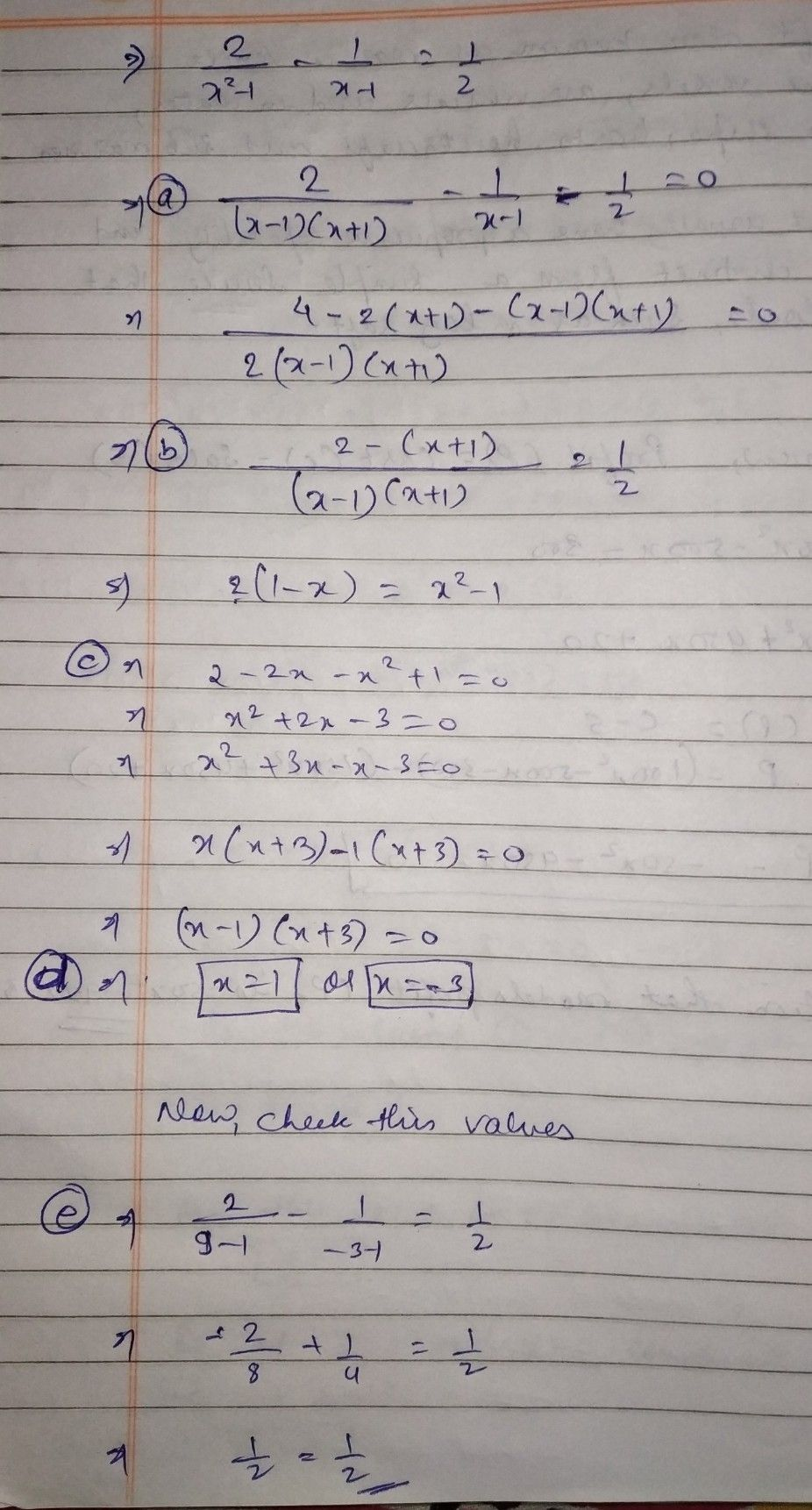Symbol
Problem1. Solve example 2 of the nitional'equation by following the given steps. $xan71$ I Example 2 Rational Equation $\dfrac {x-3} {x^{2-25}}+\dfrac {1} {x+5}=\dfrac {1} {\left(x-5\right)}$ $\dfrac {2} {x-}$ $\dfrac {1} {-1}=-$ $2$ 1. Find the Lea'st $1CD$ Comnon Denominator $\left(x+5\right)\left(x-5\right)$ (LCD). 2. Multiply both sides of $\left(x+5\right)\left(x-5\right)|\dfrac {x-1} {x2-26}+\dfrac {1} {x45}=$ the cquation tby its the $CD$ $-1$ 3. Apply the Distribulive $\left(x-3\right)+1\left(x-5\right)=16x+5\right)$ Property and then $x-3+x-5=x+5$ simplify. simplify: $2x-0-x-5$ $2x-x=y-5$ $x=13$ 4. Find all the possible $x=13$ 1zthues of x. 5. Check euch value by substituting into original cruation and rejeet any extruncous $500113$ $3^{°-5}$ $-0$ 1
10th-13th grade
Algebra
Search count: 112
SolutionQanda teacher - mamta